﻿ Equilibrium, Kinetic and Thermodynamics of Biosorption of Lead (II) Copper (II) and Cadmium (II) Ions fromAqueous Solutions ontoOlive Leaves Powder Scientific & Academic Publishing: The article detailed informationAmerican Journal of Chemistry

2012;  2(4): 238-244

doi: 10.5923/j.chemistry.20120204.09

### Equilibrium, Kinetic and Thermodynamics of Biosorption of Lead (II) Copper (II) and Cadmium (II) Ions fromAqueous Solutions ontoOlive Leaves Powder

Akl M. Awwad 1, Ahlam M. Farhan 2

1Royal Scientific Society, Princess Sumaya University for Technology, El Hassan Science City, Amman, Jordan

2Department of Chemistry, College of Science for women, University of Baghdad, Baghdad, Iraq

Correspondence to: Akl M. Awwad , Royal Scientific Society, Princess Sumaya University for Technology, El Hassan Science City, Amman, Jordan.
 Email: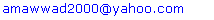Abstract

Biosorption of lead (II), copper (II) and cadmium (II) ions from aqueous solutions onto olive leaves powder has been investigated. The biosorption of lead (II), copper (II), and cadmium (II) was found to be dependent on solution pH, initial metal ion concentrations, biosorbent dose, contact time and temperature. The experimental equilibrium biosorption data were analyzed by two widely used two-parameters, Langmuir and Freundlich isotherm models. The Langmuir model gave a better fit than the Freundlich model. The kinetic studies indicated that the biosorption process of the metal ions followed well pseudo-second-order model. The thermodynamic parameters Gibbs free energy, ∆G, enthalpy, ∆H, and entropy, ∆S were also calculated, and the values indicated that the biosorption process was endothermic and spontaneous in nature. It was concluded that olive leaves powder can be used as an effective, low cost, and environmentally friendly biosorbent for removal of Pb(II), Cu(II) and Cd(II) ions from aqueous solution.

### 1. Introduction

Heavy metal ions are present in the wastewater of several industries, such as metal cleaning and plating baths, refineries, paper and pulp, fertilizer, and wood preservatives.Different methods have been used on metal content reduction from water and industrial waste such as chemical precipitation, ion exchange, membrane filtration, electrolytic methods, reverse osmosis, solvent extraction and activated carbon adsorption[1-6]. These conventional techniques can reduce metal ions, but they do not appear to be highly effective due to the limitations in the pH range as well as the high material and operational costs. Among these various treatment techniques, activated carbon adsorption is one of the most commonly used due to its high efficiency and easy operation.In recent years, considerable attention has been focused on removal of metal ions from aqueous solution using adsorbents derived from low-cost agro-wastes[8-16].
In the present work,we have studied the potential oflead (II), copper (II) , and cadmium (II) biosorption on a agro material which olive leaves (OL) coming from olive tree waste.Results from this study can be used to assess the utility of live leaves powder (OL)for lead (II), copper (II), and cadmium (II) removal from water and industrial wastewaters.

### 2. Experimental

The raw olive leaves (OL) was collected from a local plantation. This agricultural waste was thoroughly rinsed with water to remove dust and soluble material. Then it was allowed to dry at room temperature. The dried waste was grounded to a fine powder in a grinding mill (Retsch RM 100) and sieved to get size fraction < 44 µm, and then dried in an oven at 60 ℃ for 24 h.

#### 2.2. Materials

All chemicals used were of analytical reagent (AR) grade. Stock solutions of 1000 mg/L of lead (II), copper (II) and cadmium (II) were prepared from nitrates oflead, copper and cadmium which was purchased from Fluka AG using double distilled water. Desired test solutions of metalions were prepared using appropriate subsequent dilutions of the stock solution. The range of concentrations of metal ions prepared from standard solution varies between 10 and 100 mg/L. Before mixing the adsorbent, the pH of each test solution was adjusted to the required value with 0.1 M NaOH or 0.1 M HCl.

#### 2.3. Analysis

The concentrations ofPb (II),Cd(II) and Cu(II) ions in the solutions before and after equilibrium were determined by AAS6300 Atomic absorption spectrometer (Shimadzu, Japan). The pH of the solution was measured with a WTW pH meter using a combined glass electrode. Fourier Transform Infrared Spectroscopy, FTIR (IR Prestige-21, Shimadzu, Japan) was used to identify the different chemical functional groups present in the olive leaves powder (OL). FTIR analyses also used to determine the functional groups which are responsible for the metal binding with OL. The analysis was carried out usingKBr and the spectral range varying from 4000 to 400 cm−1.

#### 2.4. Biosorption Experiments

Batch biosorption experiments were conducted by mixing biosorbent with metal ion solutions with desired concentration in 250 mL glass flask. The glass flasks were stoppered during the equilibration period and placed on a temperature controlled shaker at a speed 120 r/min.The effect of pH on the equilibrium biosorption of metal ions was investigated by mixing.The amount of biosorption was calculated based on the difference between the initial (Co, mg/L) and final concentration (Ce, mg/L) in every flask, as follows: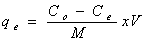(1)
where qe is the metal uptake capacity (mg/g), V the volume of the metal solution in the flask (L) and M is the dry mass of biosorbent (g).

### 3. Results and Discussion

#### 3.1. FT-IR Analysis

To investigate the functional groups of OL and copper loaded OL, a FT-IR study was carried out and the spectra are shown in fig. 1 and 2.The OL leaves displays a number of absorption peaks, reflecting the complex nature of the OL. A strong and broad peak at 3367 cm−1 results due to the stretching of the N–H bond of amino groups and indicative of bonded hydroxyl group of alcohols and phenols. A change in peak positionto 3298 cm-1in the spectrum of the copper loaded OL, fig.2, indicates the binding of copper with amino and/ or hydroxyl groups. The strong absorption peak at 2935 cm−1 and 22916 cm−1 could be assigned to –CH stretching vibrations of –CH3 and –CH2 functional groups. The shoulder peak in pure olive leave powder at 1735 cm-1 is shifted to lower frequency 1701 cm-1 due to the involvement of carbonyl –CO group from carboxylic acids in the biosorption process of copper ions with olive leave powder. The peak at 1654 cm−1 indicates the fingerprint region of CO, C–O and O–H groups, which exists as functional groups of OL and shifting of this peak to 1604 cm−1, indicated involvement of these groups in copper binding. The region between 1462 and 1000 cm-1 is the fingerprint region, OH, and C-H bending vibration and C-O stretching vibration absorption bands The absorption peaks at 1327,and 1088 cm−1 could be attributed to the presence of C–O stretching,. The intense band at 1088 cm-1 can be assigned to the C-O of alcohols and carboxylic acids. The shift of the peak from 1083 to 1014 cm−1 also suggests the involvement of C–O group in binding Cu (II). The shifts in the absorption peaks generally observed indicate the existence of a copper binding process taking place on the surface of the olive leaves powder.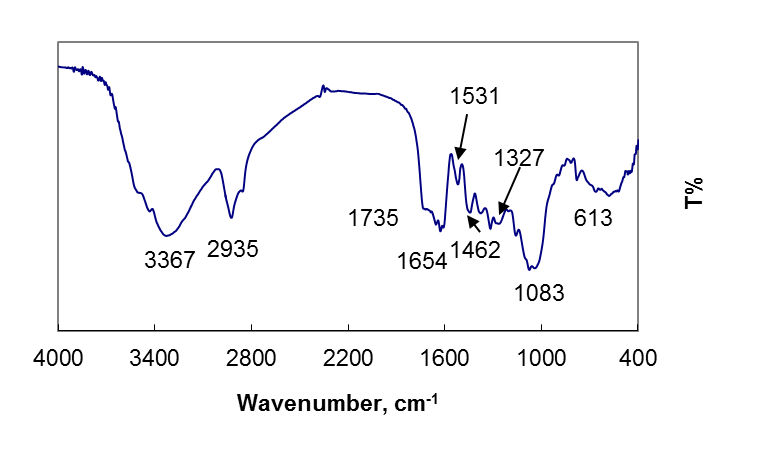Figure 1. FT-IR of olive leaves powderFigure 2. FT-IR of olive leaves powder loaded with Cu (II) ions

#### 3.2. Effect of pH

The pH has been identified as one of the most important parameter that is effective on metal sorption. It is directly related with competition ability of hydrogen ions with metal ions to active sites on the biosorbent surface. Generally, metal biosorption involves complex mechanisms of ion exchange, chelation, adsorption by physical forces, and spaces of the cell structural network of a biosorbent. The FTIR spectroscopic analysis showed that the olive leaves powder has a variety of functional groups, such as carboxyl, hydroxyl and amine and these groups are involved in almost all potential binding mechanisms. Moreover, depending on the pH values of the aqueous solutions these functional groups participate in metal ion bindings. The effect of pH on the biosorption of Pb(II), Cu(II) and Cd(II) ions onto olive leaves powder was studied at pH 1.0–8.0. The maximum biosorption was observed at pH 4.0, 6.0 and 5.0 for Pb(II), Cu(II) and Cd(II), respectively. Therefore, the remaining all biosorption experiments were carried out at this pH values. The biosorption mechanisms on the olive leaves powder surface reflect the nature of the physicochemical interaction of the solution. At highly acidic pH (pH < 1.0), the overall surface charge on the activesites became positive and metal cations and protons complete for binding sites on cell wall, which results in lower uptake of metal. The biosorbent surface was more negatively charged as the pH solution increased from 1.0 to 6.0. The functional groups of the olive leaves powder were more deprotonated and thus available for the metal ions. Decrease in biosorption yield at higher pH (pH > 6) is not only related the formation of soluble hydroxylated complexes of the metal ions (lead ions in the form of Pb(OH)2, copperions in form of Cu(OH)2, and cadmium ions in the form of Cd(OH)2) but also to the ionized nature of the cell wall surface of the olive leaves powder under the studied pH.

#### 3.3. Effect of Contact Time

The rate of biosorption is important for designing batch biosorption experiments. Therefore, the effect of contact time on the biosorption of Pb(II), Cd(II) and Cu(II) was investigated. The biosorption yield of Pb(II), Cd(II) and Cu(II) increased considerably until the contact time reached 120 min.Further increase in contact time did not enhance the biosorption, so, the optimum contact time was selected as 120 min for further experiments

#### 3.4. Effect of Adsorbent Dose on Biosorption

The biosorbent dosage is an important parameter because this determines the capacity of a biosorbent for a given initial concentration. The biosorption efficiency for Pb(II), Cd(II) and Cu(II) ions as a function of biosorbent dosage was investigated. The percentage of the metal biosorption steeply increases with the biosorbent loading up to 0.5 g/0.1 L. This result can be explained by the fact that the biosorption sites remain unsaturated during the biosorptionreaction whereas the number of sites available for biosorption site increases by increasing the biosorbent dose. Moreover, the maximum biosorption, 74.9% for Cu(II), 85.2% for Cd(II) and 94.5% for Pb(II), ions was attained at biosorbent dosage, 0.5 g/0.1 L. Therefore, the optimum biosorbent dosage was taken as 0.5 g/0.1 L for further experiments.

#### 3.5. BiosorptionIsotherms

An adsorption isotherm describes the fraction of sorbate molecules that are partitioned between liquid and solid phases at equilibrium. Adsorption of metal ions onto OL particles was modeled using adsorption isotherms.
##### 3.5.1. FreundlichIsotherm
The Freundlich isotherm model is the well-known earliest relationship describing the adsorption process. This model applies to adsorption on heterogeneous surfaces with the interaction between adsorbed molecules and the application of the Freundlich equation also suggests that sorption energy exponentially decreases on completion of the sorption centers of an adsorbent. This isotherm is an empirical equation and can be employed to describe heterogeneous systems and is expressed as follows in linear form: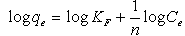(2)
whereKF is the Freundlich constant related to the bonding energy. 1/n is the heterogeneity factor and n (g/L) is a measure of the deviation from linearity of adsorption. Freundlich equilibrium constants were determined from the plot oflog qeversus log Ce,fig. 3 on the basis of the linear of Freundlich equation (2).The nvalue indicates the degree of non-linearity between solution concentration and adsorption as follows: if n = 1, then adsorption is linear; if n < 1, then adsorption is a chemical process; if n > 1, then adsorption is a physical process. The n value in Freundlich equation was found to be 1.45-1.59 for OL, Table 1.Since n lie between 1 and 10, this indicate the physical biosorption of copper (II) onto OL. The values of regression coefficients R2 are regarded as a measure of goodness of fit of the experimental data to the isotherm models.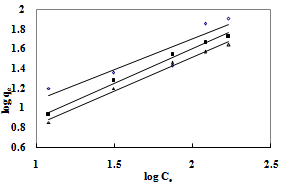Figure 3. Freundlich adsorption isotherm of ◊, Pb (II); ■, Cd (II); ∆, Cu(II) onto OL at303 K
##### 3.5.2. Langmuir Isotherm
The Langmuir isotherm assumes monolayer adsorption on a uniform surface with a finite number of adsorption sites. Once a site is filled, no further sorption can take place at that site. As such the surface will eventually reach a saturation point where the maximum adsorption of the surface will be achieved. The linear form of the Langmuir isotherm model is described as: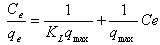(3)
where KL is the Langmuir constant related to the energy of adsorption and qmax is the maximum adsorption capacity (mg /g). Values of Langmuir parameters qmax and KL were calculated from the slope and intercept of the linear plot of Ce/qe versus Ceas shown in fig. 4. Values of qmax, KLand regression coefficient R2 are listed in Table 1. These values for OL biosorbent indicated that Langmuir theory describes the biosorption phenomena favarouble.The essential characteristics of the Langmuir isotherm parameters can be used to predict the affinity between the sorbate and sorbent using separation factor or dimensionless equilibrium parameter, RL expressed as in the following equation: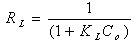(4)
where KL is the Langmuir constant and Co is the initial concentration of copper (II) ions. The value of separation parameter RL provides important information about the nature of adsorption. The value of RL indicated the type of Langmuir isotherm to be irreversible (RL=0), favorable (0<RL<1), linear (RL=1) or unfavorable (RL>1). The RL was found to be 0.64-0.88 for concentration of 10–100 mg/L of metal ions. They are in the range of 0-1 which indicates the favorablebiosorption.
 Table 1. Langmui andFreundlich constants for biosorption of Cd(II) onto olive leaves powderat different temperatures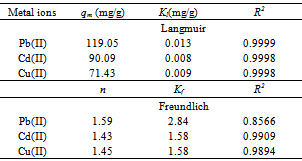Parameters from two kinetic models, pseudo first-orderand pseudo second-order were fit to experimental data to examine the adsorption kinetics of copper (II) uptake by OL.
##### 3.6.1. Pseudo First-Order Kinetics
The pseudo-first order equation of Lagergrenis generally expressed as follows: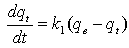(5)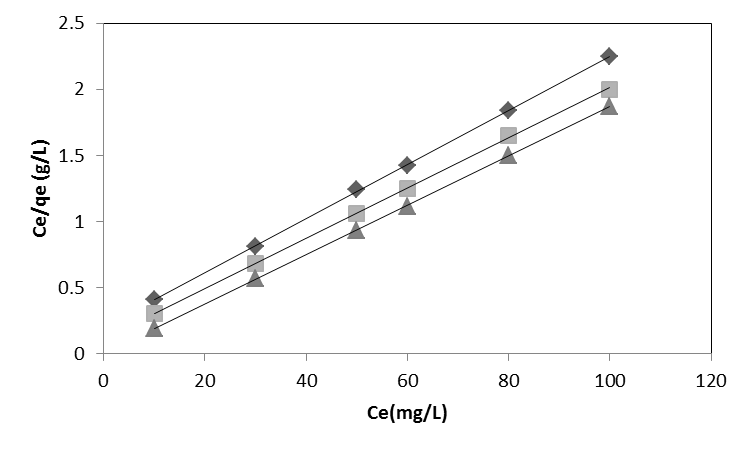Figure 4. Langmuir adsorption isotherms for ♦, Pb(II); ■,Cd (II); ∆, Cu(II) ions onto OL at303 K
Whereqeand qt are the sorption capacities at equilibrium and at time t, respectively (mg/g) and k1 is the rate constant of pseudo-first order sorption, (1/min). After integration and applying boundary conditions, qt = 0 to qt = qtat t = 0to t = t ; the integrated form of equation (5) becomes: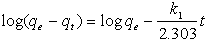(6)
The equation applicable to experimental results generally differs from a true first order equation in two ways:the parameter k1(qe - qt) does not represent the number of available sites; and the parameter log qeis an adjustable parameter which is often not found equal to the intercept of a plot of log (qe –qt) against t, whereas in a true first order sorption reactionlog qe should be equal to the intercept oflog(qeqt) against t.In order to fit equation (6) to experimental data, the equilibrium sorption capacity, qe must be known. In many cases is unknown and as chemisorption tends to become un measurably slow, the amount sorbed is still significantly smaller than the equilibrium amount. In most cases in the literature, the pseudo-first order equation of Lagergren does not fit well for the whole range of contact time and is generally applicable over the initial 20 to 60 minutes of the sorption process. Furthermore, one has to find some means of extrapolating the experimental data to t = ∞, on treating qeas an adjustable parameter to be determined by trial and error. For this reason, it is therefore necessary to use trial and error to obtain the equilibrium sorption capacity, in order to analyze the pseudo-first order model kinetics.
The pseudo first order rate constant can be obtained from the slope of plot between log (qeqt) against time, t.fig. 5 shows the Lagergren pseudo-first order kinetic plot for the adsorption of metalions onto OL powder. The calculated values and their corresponding linear regression correlation coefficient values are shown in Table 2. The linear regression correlation coefficient value found 0.8764, 0.9807 and 0.9007 for Pb(II), Cd(II) and Cu(II) ions , respectively which shows that this model cannot be applied to predict the adsorption kinetic model.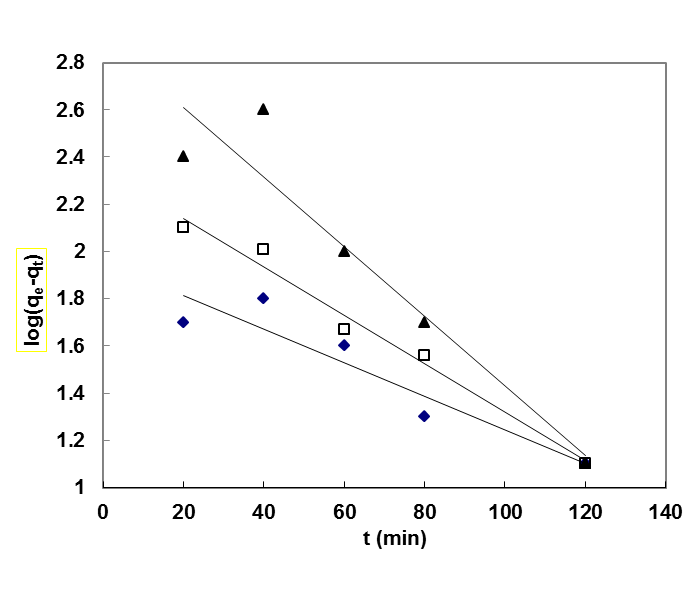Figure 5. Pseudo-first order kinetic model for ♦, Pb(II); □, Cd(II); ▲, Cu(II) biosorption onto OL at303 K
##### 3.6.2. Pseudo-Second Order Kinetics
The pseudo second-order rate expression, which has been applied for analyzing chemisorption kinetics rateis expressed as: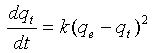(7)
Where qeand qt are the sorption capacity at equilibrium and at time t, respectively (mg/g) and k is the rate constant of pseudo-second order sorption, (g/mg min). For the boundary conditions to qt = 0 to qt = qt at t = 0 to t = t; ; the integrated form of equation (7 ) becomes: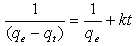(8)
Equation (8) can be rearranged to obtain: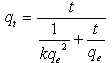(9)
The linear form of equation (9)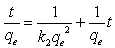(10)
Where t is the contact time (min), qe (mg/g) and qt (mg/g) are the amount of the solute adsorbed at equilibrium and at any time, t. If pseudo-second order kinetics is applicable, the plot of t/qt versus t of equation (10) should give a linear relationship, from which qe and k can be determined from the slope and intercept of the plot, fig.6. The pseudo-second order rate constant k, the calculated qe value and the corresponding linear regression correlation coefficient value are given in Table 2. At all initial metal concentrations, the linear regression correlation coefficient R2 values were higher. The higher values confirm that the adsorption data are well represented by pseudo-second order kinetics.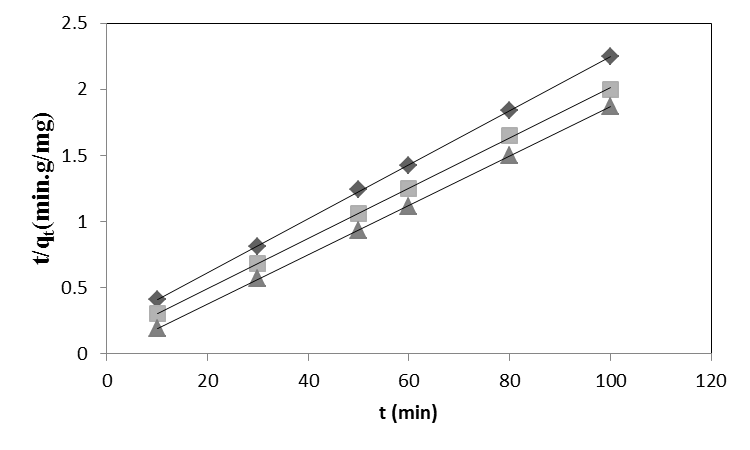Figure 6. Pseudo-second order kinetic model for ♦, Pb(II); ■, Cd (II); ▲, Cu(II) biosorption onto OL at 303 K
 Table 2. Biosorption kinetic model for metal ionsonto OL at 303 K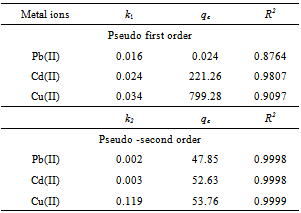#### 3.7. Thermodynamic Parameters

In order to describe thermodynamic behaviour of the biosorption of Pb(II), Cd(II) and Cu(II) ions onto olive leavespowder, thermodynamic parameters including the change in free energy (∆G◦), enthalpy (∆H◦) and entropy (∆S◦) were calculated from following equations: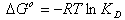(11)
where R is the universal gas constant (8.314×103 kJ/mol K), T is the temperature
(K) and KD is the distribution coefficient.
The thermodynamic equilibrium constant (KD) of the adsorption is defined as: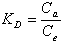(12)
where, Ca is mg of adsorbate adsorbed per liter and Ce is the equilibrium concentration of solution, mg/L. According to thermodynamics, the Gibb’s free energy change is also related to the enthalpy change (∆H◦) and entropy change (∆S◦) at constant temperature by the van’t Hoff equation: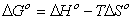(13)
Equations (11) and (13) can be written as: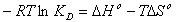(14)
or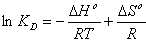(15)
According to Eq. 15, the values of enthalpy change (∆H◦)and entropy change (∆S◦) werecalculated from the slope and intercept of the plot of ln KD vs. 1/T, Fig 7.The calculated values of thermodynamic paramours ∆G◦, ∆H◦, and ∆S◦ for the biosorption of Pb(II), Cd(II) and Cu(II) onto olive leaves powder are reported in Table 3. A positive value of H◦as 17.76, 17.38 and 18.14 kJ/mol for lead, cadmium and copper removal with OL, indicated the endothermic nature of the process. A negative value of the free energy (G◦)[-2.66, -2.38 and -2.07 kJ/ molfor Pb(II), Cd(II) and Cu(II), respectively] at 293 K indicated the spontaneous nature of the biosorptionprocess. It was also noted that the change in free energy, increases with increase in which exhibits an increase in adsorption with rise in temperature. This could be possibly because of activation of more sites on the surface of OLwith increase in temperature or that the energy of biosorption sites has an exponential distribution and a higher temperature enables the energy barrier of biosorption to be overcome. Thephysical adsorption the free energy change (G◦) ranges from (−20 to 0) kJ/ mol and for chemical adsorption it ranges between (−80 and −400) kJ mol1. The G◦ for Pb(II), Cd(II) and Cu(II) biosorption onto OL was in the range of (−2.07 to −4.06) kJ mol1 and so the adsorption was predominantly physical biosorption.A positive value of S◦ as 69.63 J/ mol K showed increased randomness at solid solution interface during the adsorption of Pb(II), Cd(II) and Cu(II) ions onto olive leaves powder.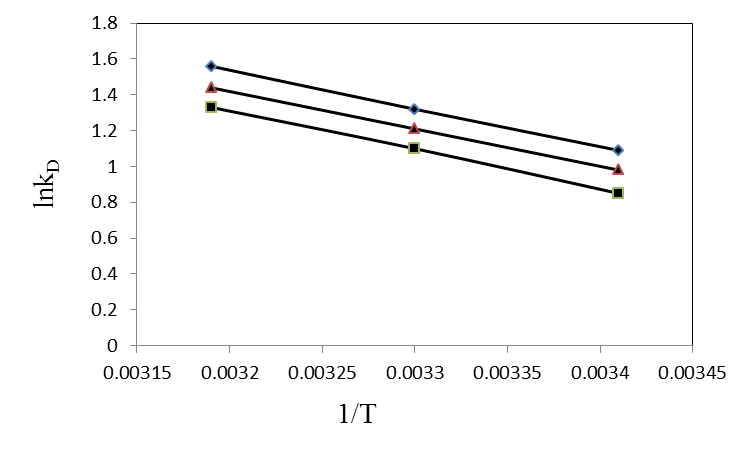Figure 7. Plot of ln KD for biosorption of ♦, Pb(II); ▲, Cd(II); ■, Cu(II) onto olive leaves powder
 Table 3. Thermodynamic parameters for biosorption of Pb(II), Cd(II) and Cu(II) onto olive leaves powder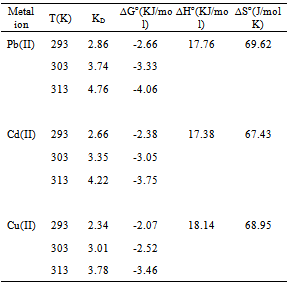#### 3.8. Desorption and Regeneration Studies

In order to investigate biosorption on metal ions from copper- loaded OL, the copper–loaded biosorbent was treated with HCl. Desorption studies were performed with different hydrochloric acids concentration. Complete elution of the loaded metal 98.44% could be achieved by using 0.1N HCl. To keep the biosorption process cost down, our results indicate that OL biosorbent could be used repeatedly in Cu (II)biosorption.

### 4. Conclusions

The potential ofoliveleaves (OL)powder for the removal of Pb(II), Cu (II)and Cd(II) ions from aqueous solutions and wastewaterwas dependent on biosorption process such as solution pH, initial metal ions concentration, biosorbent dose, contact time and temperature.The Langmuir biosorption isothermswere demonstrated to provide the best correlation for the biosorption of Pb(II), Cd(II) and Cu(II)ionsonto OL. The kinetic results provided the best correlation of the experimental data of biosorption of metal ions onto OL by pseudo second-order equation. Thermodynamic parameters of the biosorption confirmed the endothermic nature of sorption process as did the positive heat of enthalpy ∆Ho, accompanied by a positive value of entropy change ∆So. The standard Gibb’s free energy change ∆Go during adsorption process was negative, corresponding to feasible, spontaneous biosorption. it can be concluded that since the OL is an easily, locally available, low-cost adsorbent and has a considerable high biosorption capacity, it may be treated as an alternative adsorbent for treatment of wastewater containing lead (II), copper(II) and cadmium (II)ions.

### ACKNOWLEDGEMENTS

Authors are thankful for Cardiff School of Chemistry, Cardiff University, and Royal Scientific Society for providing the necessary facilities to carry out this work.